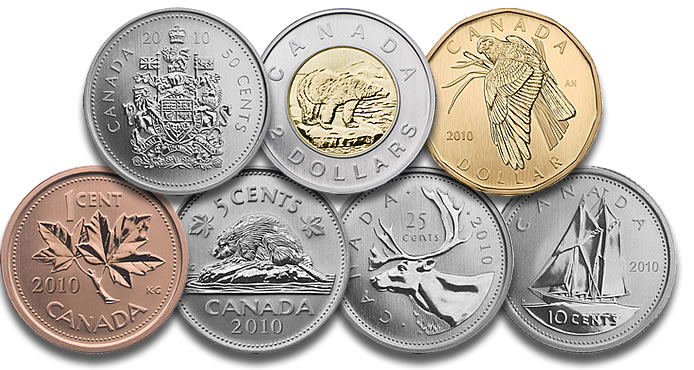## Problem 4: ChangeYour friend is just getting into competitive programming, and is trying to solve the classic "change" problem. You'll give him a target value K (1 ≤ K ≤ 109) and some distinct coin denominations (each of which is between 1 and K, inclusive), and he'll try to determine whether or not a total of K can be produced using a set of (possibly duplicate) coins having only those denominations. The algorithm he's come up with to do so is greedy - starting from an empty set of coins, it repeatedly adds on the largest possible coin which won't cause the total to exceed K, until it either reaches a total of K, or is unable to add on any more coins and gives up.

You're not sure what coin denominations you'd like to give him, but there are N (0 ≤ N ≤ 2000) distinct denominations which you definitely don't want to include. The i-th of these is Di (1 ≤ DiK).

Your friend is convinced that his so algorithm is so good that he'll be able to attain a total of K no matter what denominations he's given! This is quite the bold claim. You'd like to prove him wrong by choosing a (possibly empty) set denominations such that his algorithm will fail to reach a total of K using them. Note that it doesn't matter whether or not a more correct algorithm would succeed, as long as your friend's greedy algorithm fails to achieve a total of K.

Clearly, one option would be to simply give your friend 0 denominations to use. However, that's too easy - you'd like to prove as convincingly as possible that their algorithm is sub-par. As such, you'd like to determine the maximum number of distinct denominations which you can give your friend, such that their algorithm will still fail to reach a total of K using them.

In test cases worth 6/37 of the points, K ≤ 20.
In test cases worth another 20/37 of the points, K ≤ 1000.

### Input Format

The first line of input consists of two space-separated integers, K and N.
N lines follow, the i-th of which consists of a single integer, Di, for i = 1..N.

### Output Format

Output a single integer, the maximum number of distinct denominations which you can give your friend.

```7 4
3 7 6 1
```

```2
```

```4 1
3
```

```0
```

### Sample Explanation 2

In the first case, one possibility is to give your friend the set of denominations (2, 4}. His algorithm would use a 4 coin followed by a 2 coin, and then give up.

In the second case, you must resort to giving your friend no denominations, as his algorithm would achieve a total of 4 if given any non-empty subset of the denominations {1, 2, 4}.

Point Value: 16 (partial)
Time Limit: 2.00s
Memory Limit: 64M